# Describing Chemical Reactions Chemical Reactions A chemical reaction

• Slides: 21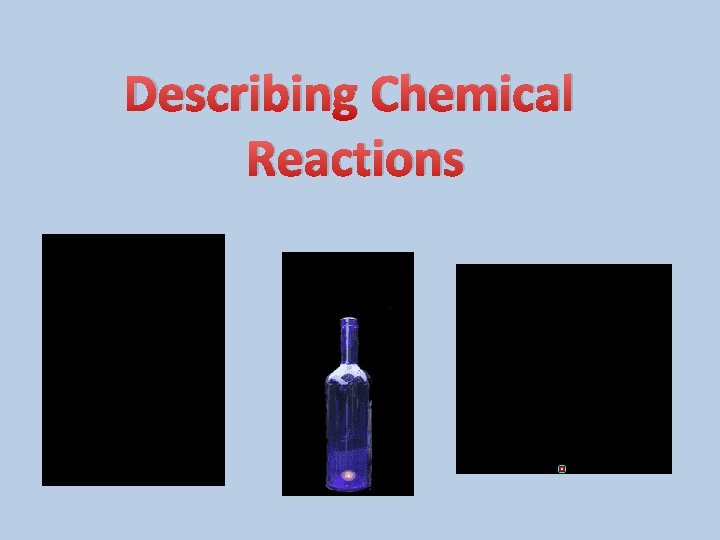Describing Chemical Reactions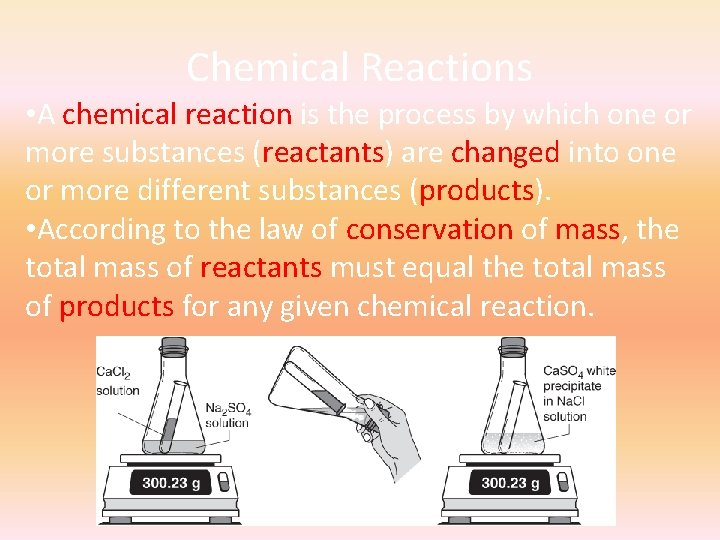Chemical Reactions • A chemical reaction is the process by which one or more substances (reactants) are changed into one or more different substances (products). • According to the law of conservation of mass, the total mass of reactants must equal the total mass of products for any given chemical reaction.• Chemical reactions are described by chemical equations. • A chemical equation represents, with symbols and formulas, the identities and relative molecular or molar amounts of the reactants and products in a chemical reactions. • Example: the following chemical equation shows that the reactant ammonium dichromate yields the products nitrogen, chromium(III) oxide, and water.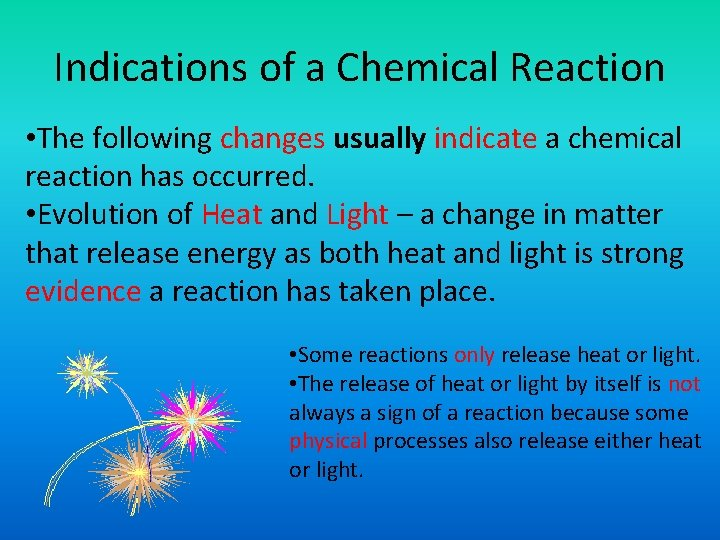Indications of a Chemical Reaction • The following changes usually indicate a chemical reaction has occurred. • Evolution of Heat and Light – a change in matter that release energy as both heat and light is strong evidence a reaction has taken place. • Some reactions only release heat or light. • The release of heat or light by itself is not always a sign of a reaction because some physical processes also release either heat or light.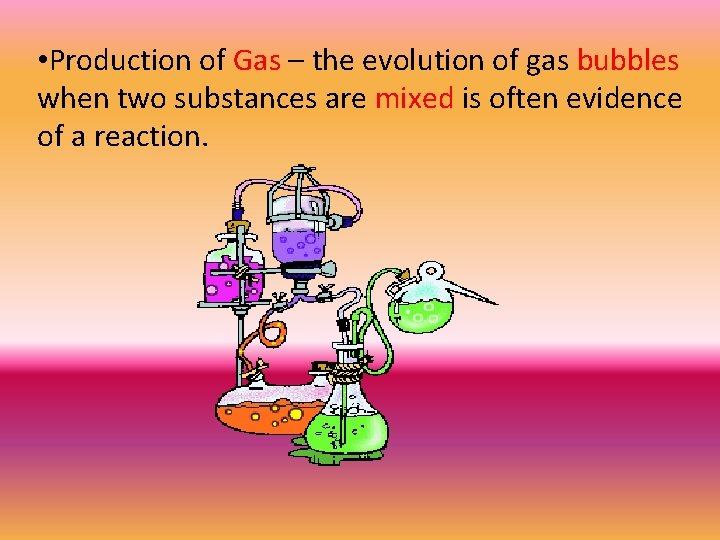• Production of Gas – the evolution of gas bubbles when two substances are mixed is often evidence of a reaction.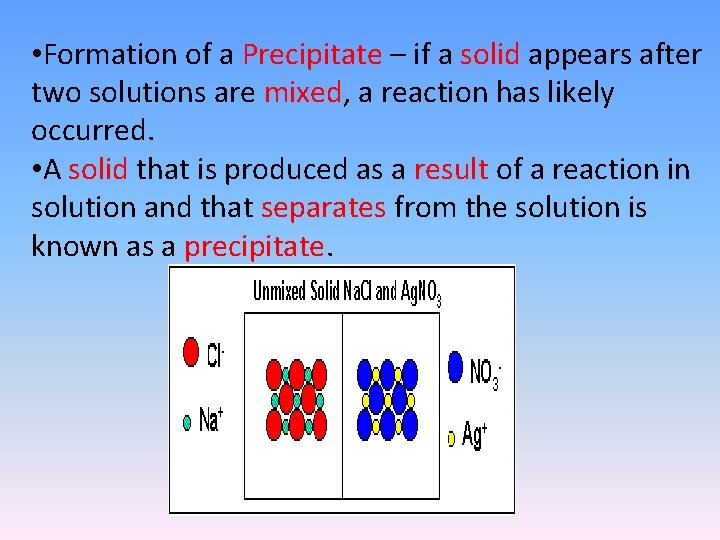• Formation of a Precipitate – if a solid appears after two solutions are mixed, a reaction has likely occurred. • A solid that is produced as a result of a reaction in solution and that separates from the solution is known as a precipitate.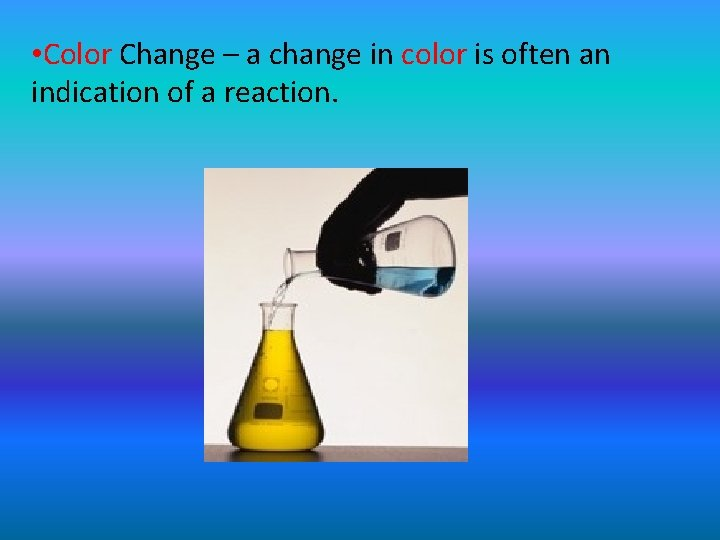• Color Change – a change in color is often an indication of a reaction.Characteristics of Chemical Equations • The equation must represent known facts. All reactants and products must be identified. • The equation must contain the correct formulas for the reactants and products. • The law of conservation of mass must be satisfied.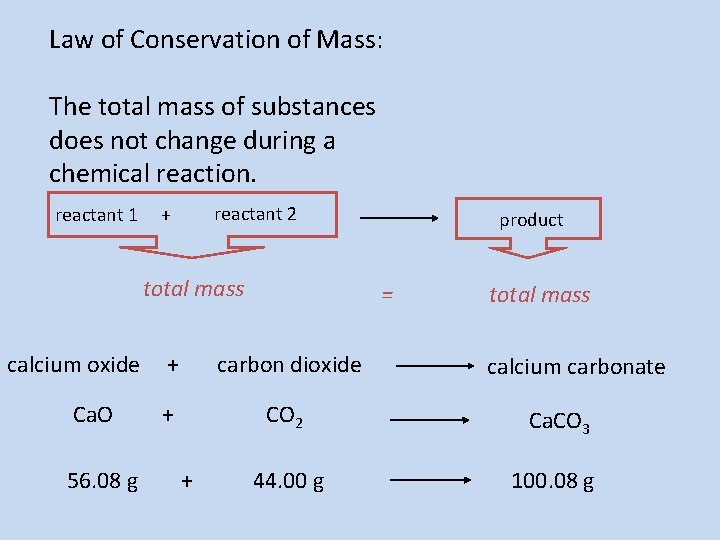Law of Conservation of Mass: The total mass of substances does not change during a chemical reaction. reactant 1 reactant 2 + total mass calcium oxide Ca. O 56. 08 g product = total mass + carbon dioxide + CO 2 Ca. CO 3 44. 00 g 100. 08 g + calcium carbonate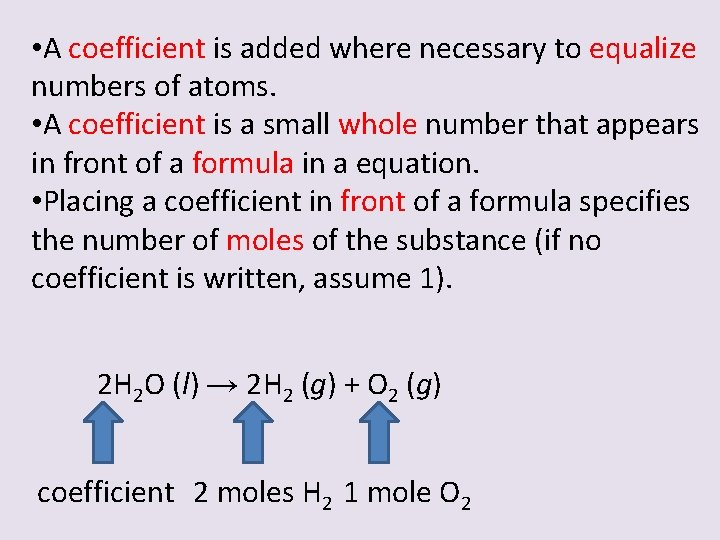• A coefficient is added where necessary to equalize numbers of atoms. • A coefficient is a small whole number that appears in front of a formula in a equation. • Placing a coefficient in front of a formula specifies the number of moles of the substance (if no coefficient is written, assume 1). 2 H 2 O (l) → 2 H 2 (g) + O 2 (g) coefficient 2 moles H 2 1 mole O 2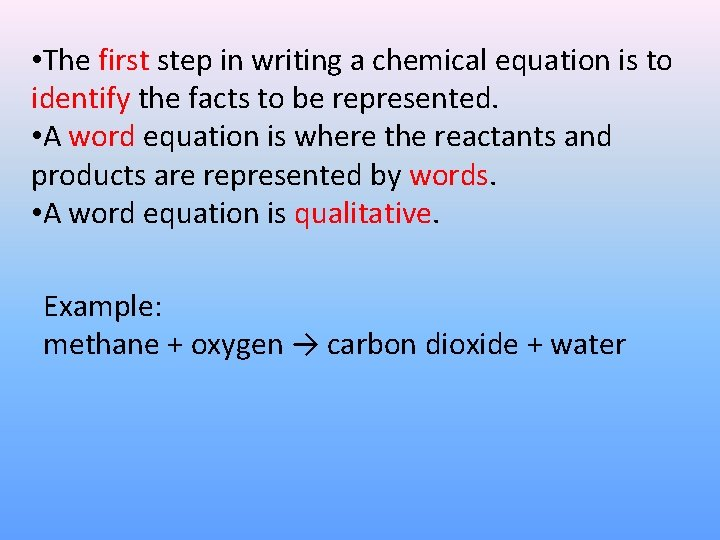• The first step in writing a chemical equation is to identify the facts to be represented. • A word equation is where the reactants and products are represented by words. • A word equation is qualitative. Example: methane + oxygen → carbon dioxide + water• The next step in writing a correct chemical equation is to replace the names of the reactants and products with appropriate symbols and formulas. • A formula equation (also qualitative) represents the reactants and products of a chemical reaction by their symbols or formulas. Example: CH 4 (g) + O 2 (g) → CO 2 (g) + H 2 O (g) (not balanced) methane + oxygen → carbon dioxide + water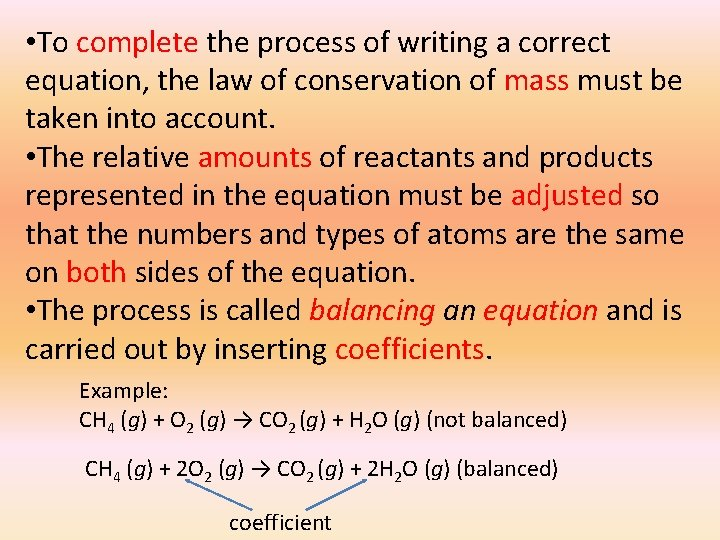• To complete the process of writing a correct equation, the law of conservation of mass must be taken into account. • The relative amounts of reactants and products represented in the equation must be adjusted so that the numbers and types of atoms are the same on both sides of the equation. • The process is called balancing an equation and is carried out by inserting coefficients. Example: CH 4 (g) + O 2 (g) → CO 2 (g) + H 2 O (g) (not balanced) CH 4 (g) + 2 O 2 (g) → CO 2 (g) + 2 H 2 O (g) (balanced) coefficient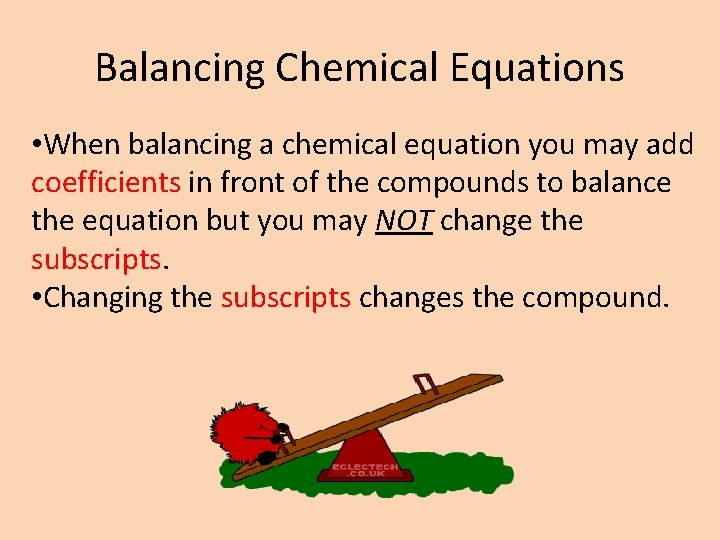Balancing Chemical Equations • When balancing a chemical equation you may add coefficients in front of the compounds to balance the equation but you may NOT change the subscripts. • Changing the subscripts changes the compound.Steps to Balancing Chemical Equations • There are four basic steps to balancing a chemical equation.Step • Write the correct formulas for the reactants and products. DO NOT TRY TO BALANCE IT YET! You must write the correct formulas first. • And most importantly, once you write them correctly DO NOT CHANGE THE FORMULAS! Aluminum sulfate and calcium hydroxide react to produce aluminum hydroxide and calcium sulfate. Al 2(SO 4)3 + Ca(OH)2 → Al(OH)3 + Ca. SO 4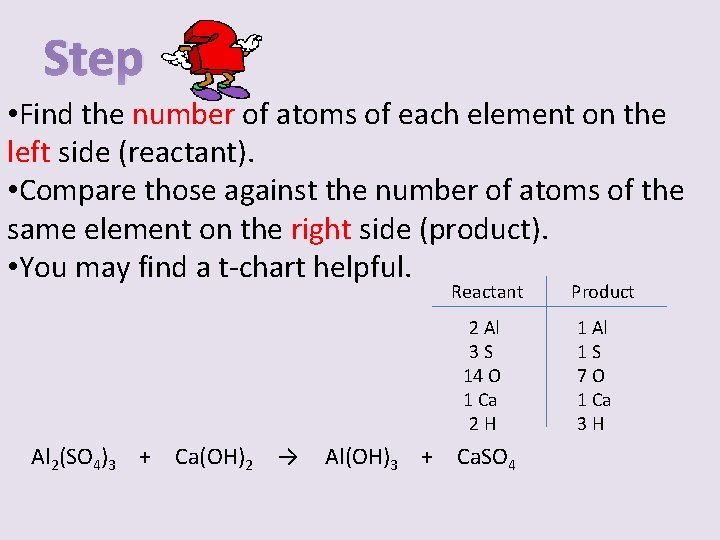Step • Find the number of atoms of each element on the left side (reactant). • Compare those against the number of atoms of the same element on the right side (product). • You may find a t-chart helpful. Reactant 2 Al 3 S 14 O 1 Ca 2 H Al 2(SO 4)3 + Ca(OH)2 → Al(OH)3 + Ca. SO 4 Product 1 Al 1 S 7 O 1 Ca 3 HStep • Determine where to place coefficients in front of formulas so that the left side has the same number of atoms as the right side for EACH element to balance the equation. Reactant 2 Al 3 S 14 O 18 1 Ca 3 2 H 6 Al 2(SO 4)3 + 3 Ca(OH)2 → 2 Al(OH)3 + 3 Ca. SO 4 Product 2 Al 1 31 SS 10 18 7 OO 31 Ca Ca 63 HH• Check your answer to see if: • The number of atoms on both sides of the equation are now balanced. • The coefficients are in the lowest possible whole number ratios (reduced). Reactant Product 2 Al 3 S 18 O 3 Ca 6 H Al 2(SO 4)3 + 3 Ca(OH)2 → 2 Al(OH)3 + 3 Ca. SO 4 2 Al 3 S 18 O 3 Ca 6 HHelpful Hints • Take one element at a time, working left to right except for H and O. Save H for next to last and O until last. • IF everything balances except for O, and there is not way to balance O with a whole number, double all the coefficients and try again. (Because O is diatomic as an element. • Polyatomic ions that exist on both sides of the equation should be balanced as independent unitsSymbols Used in Chemical Equations (s) or (cr) solid or crystal (l) liquid (g) gas (aq) ∆ → in aqueous solution (dissolved in water) change in “produces” or “yields” indicating result of reaction